# Permutation Diagrams

A permutation of a set is a rearrangement of all its elements. (There are many special classes of permutations, such as derangements, which are permutations that leave no element in its original position.) Here are some diagrams that represent the n! possible permutations of a set with n elements.

3 elements, 3! = 6 permutations: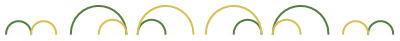4 elements, 4! = 24 permutations: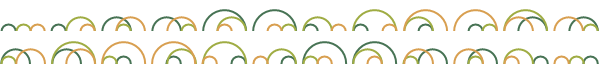5 elements, 5! = 120 permutations: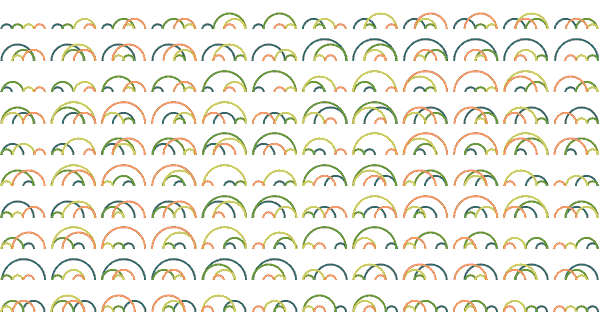6 elements, 6! = 720 permutations: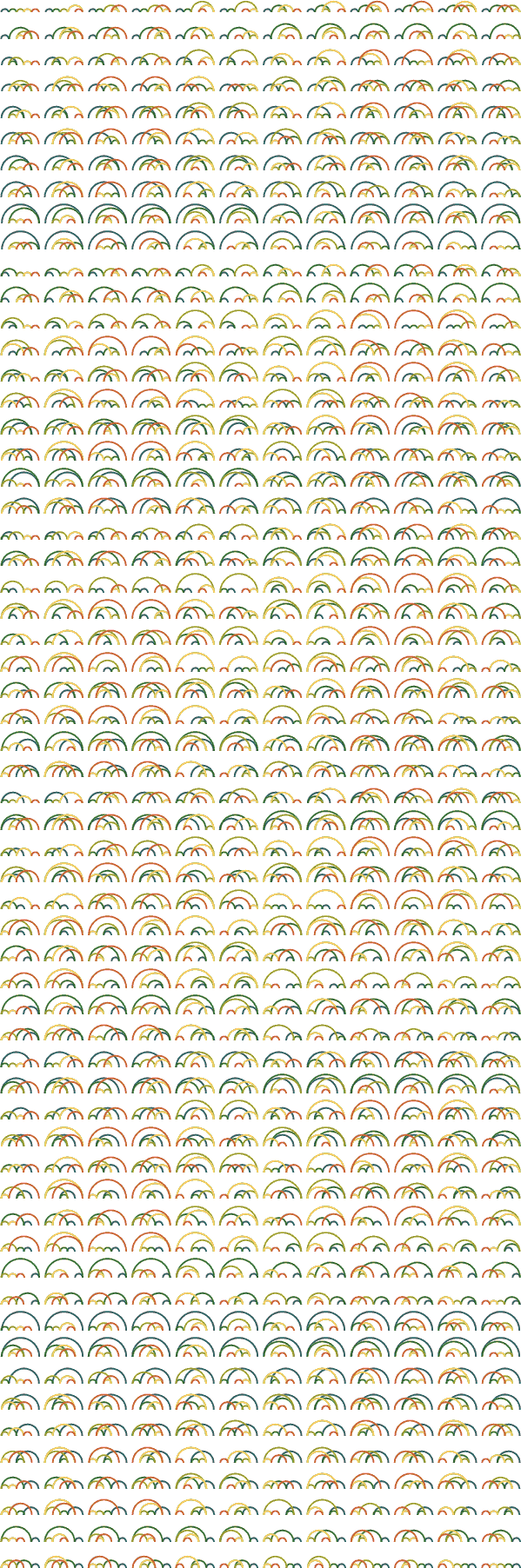The set of permutations can be partially ordered by inversions—swapping a pair of adjacent items when the left item is greater than the right—and this partial ordering can be displayed as a lattice. The following lattice shows permutations from 4321 at the top, followed by elements generated by one inversion (3421, 4231, 4312), and so on to 1234 at the bottom: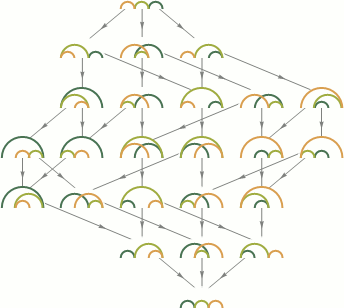Designed and rendered using Mathematica 3.0 for NeXT and Apple Macintosh and (much, much later) Mathematica 7.0 for Microsoft Windows.